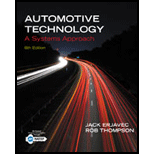# What is represented by the E, I, and R in the formula E = IR?### Automotive Technology: A Systems A...

6th Edition
Jack Erjavec + 1 other
Publisher: Cengage Learning
ISBN: 9781133612315### Automotive Technology: A Systems A...

6th Edition
Jack Erjavec + 1 other
Publisher: Cengage Learning
ISBN: 9781133612315

#### Solutions

Chapter
Section
Chapter 15, Problem 1RQ
Textbook Problem

## What is represented by the E, I, and R in the formula E = IR?

Expert Solution
To determine

The representation of E, I and R, in the formula E=IR.

### Explanation of Solution

Given:

The formula given is E = IR.

Concept used:

According to Ohm's law at a constant temperature, the voltage across a circuit is directly proportional to the current through it.

The given formula, E = IR

It is a mathematical representation of Ohm's law in which the symbol

E = voltage

I = current

R = resistance in the circuit.

Thus, the voltage in a circuit is equal to the current applied multiplied by the resistance.

### Want to see more full solutions like this?

Subscribe now to access step-by-step solutions to millions of textbook problems written by subject matter experts!

Get Solutions

### Want to see more full solutions like this?

Subscribe now to access step-by-step solutions to millions of textbook problems written by subject matter experts!

Get Solutions

Find more solutions based on key concepts
Show solutions
What does NFPA stand for?

Precision Machining Technology (MindTap Course List)

Give examples of common mechanical engineering symbols.

Engineering Fundamentals: An Introduction to Engineering (MindTap Course List)

What range of leakage current must trip an IDCI?

EBK ELECTRICAL WIRING RESIDENTIAL

Solve Problem 8.4-4 by the ultimate strength method (use the tables in Part 8 of the Manual).

Steel Design (Activate Learning with these NEW titles from Engineering!)

Which is faster: sequential or direct access? Why? (392)

Enhanced Discovering Computers 2017 (Shelly Cashman Series) (MindTap Course List)

If your motherboard supports ECC DDR3 memory, can you substitute non-ECC DDR3 memory?

A+ Guide to Hardware (Standalone Book) (MindTap Course List)